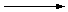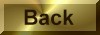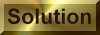Practice Problem 4

We can determine the concentration of an acidic permanganate ion solution by titrating this solution with a known amount of oxalic acid until the charactistic purple color of the MnO4- ion disappears.

H2C2O4(aq) + MnO4-(aq)CO2(g) + Mn2+(aq)

Use the half-reaction method to write a balanced equation for this reaction.

Answer

2 MnO4-(aq) + 5 H2C2O4(aq) + 6 H+(aq)10 CO2(g) + 2 Mn2+(aq) + 8 H2O(l)